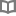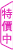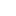經濟學的決策思想 - 喬治．史皮婁 - BOOKWALKER中文電子書

經濟學的決策思想

300年來，人類如何思索風險，選擇與不確定

作者： 喬治．史皮婁 譯者： 劉凡恩

•喬治．史皮婁（George G. Szpiro）

獲獎作家與新聞工作者，瑞士日報《新蘇黎世報》（Neue Zürcher Zeitung）長期特派員。學生時代攻讀數學、數理經濟學，之後從數學家、商管顧問轉行當記者並從事寫作。著作甚豐，包括：《數字當家》（Numbers Rule）、《定價未來》（Pricing the Future）、《數字的秘密生命：生活中最有趣的50個數學故事，像數學家一樣思考》（The Secret Life of Numbers）、《數學的秘密生命：數學家與數學大鬥智的有趣故事，課本裡沒有教的50個數學大發現》（A Mathematical Medley），《刻卜勒的猜想：「費馬最後定理」之後，最吸引人的數學問題》（Kepler's Conjecture）等。

• 放入購物車
•試閱

• 放入購物車
•試閱

• 放入購物車
•試閱• 放入購物車
•試閱• 放入購物車
•試閱• 放入購物車
•試閱• 放入購物車
•試閱• 放入購物車
•試閱

• 放入購物車
•試閱• 放入購物車
•試閱• 放入購物車
•試閱• 放入購物車
•試閱• 放入購物車
•試閱

• 放入購物車
•試閱• 放入購物車
•試閱• 放入購物車
•試閱• 放入購物車
•試閱• 放入購物車
•試閱

• 放入購物車
•試閱• 放入購物車
•試閱

• 放入購物車
•試閱

• 放入購物車
•試閱

• 放入購物車
•試閱

• 放入購物車
•無試閱• 放入購物車
•試閱

• 放入購物車
•試閱

• 放入購物車
•試閱

• 放入購物車
•試閱

• 放入購物車
•試閱

• 放入購物車
•試閱

• 放入購物車
•試閱

• 放入購物車
•試閱

• 放入購物車
•試閱

• 放入購物車
•試閱

• 放入購物車
•無試閱

• 放入購物車
•無試閱

• 放入購物車
•試閱

• 放入購物車
•試閱

• 放入購物車
•試閱

• 放入購物車
•試閱

• 放入購物車
•試閱

• 放入購物車
•試閱

• 放入購物車
•試閱

• 放入購物車
•試閱

• 放入購物車
•試閱• 放入購物車
•試閱